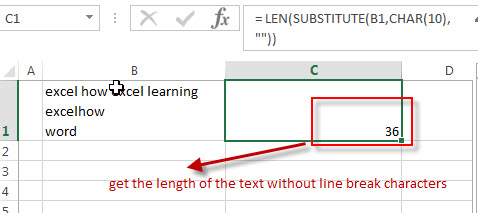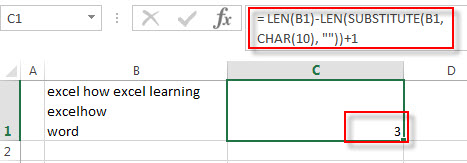# How to count the number of line breaks in a cell

This post explains that how to count the number of line breaks in a cell using excel formula. How do I count line breaks in a single cell in excel. In the previous post, we talked that how to count specific words in a cell and this post will guide you how to count line breaks characters in a text in a cell.

## Count line breaks in a cell

If you want to count the number of line breaks characters in a cell, you need to create an excel formula based on the LEN function, the SUBSTITUTE function and the CHAR function.

You need to use the SUBSTITUTE function to remove all line break characters in the text in a cell, then using the LEN function to get the length of the text without line break characters. The returned number is then subtracted from the length of the original text in a cell. And then add 1 to get the number of line breaks in the text.

Assuming that you want to count line breaks in the cell B1, you can write down an excel formula as follows:

`= LEN(B1)-LEN(SUBSTITUTE(B1,CHAR(10), ""))+1`

Let’s see how this formula works:

= LEN(SUBSTITUTE(B1,CHAR(10), “”))This formula will replace all line break characters with empty character in the text in cell B1. And then the number goes into the LEN function to get the length of the text without line break characters.

= LEN(B1)-LEN(SUBSTITUTE(B1,CHAR(10), “”))+1This formula will get the number of line break characters in text in a cell.

### Related Formulas

• count specific words in a cell or a range
If you want to count the number of a specific word in a single cell, you need to use the SUBSTITUTE function to remove all that certain word in text string, then using LEN function to calculate the length of the substring that without that specific word.…
• Count the number of words in a cell
If you want to count the number of words in a single cell, you can create an excel formula based on the IF function, the LEN function, the TRIM function and the SUBSTITUTE function. ..

### Related Functions

• Excel Substitute function
The Excel SUBSTITUTE function replaces a new text string for an old text string in a text string.The syntax of the SUBSTITUTE function is as below:= SUBSTITUTE  (text, old_text, new_text,[instance_num])….
• Excel LEN function
The Excel LEN function returns the length of a text string (the number of characters in a text string).The syntax of the LEN function is as below:= LEN(text)…
• Excel CHAR function
The Excel CHAR function returns the character specified by a number (ASCII Value).The CHAR function is a build-in function in Microsoft Excel and it is categorized as a Text Function. The syntax of the CHAR function is as below:=CHAR(number)….

### This post currently has 2 responses

•Michael says:

hi excelhow,

in case above, how to know the number of line that contains “word” ?

other case :
ab cde
fg hij
kl mno

how to know number of line that contains “hij” ?

Thanks

•scott says:

Hi Michael,
If you want to get the number of line that contains a gvien text string(such as: hij), you can try to use the below formula:
=( LEN(B1)-LEN(SUBSTITUTE(B1,”hij”, “”)))/LEN(“hij”)

-scott

Sidebar